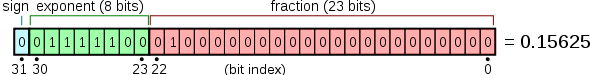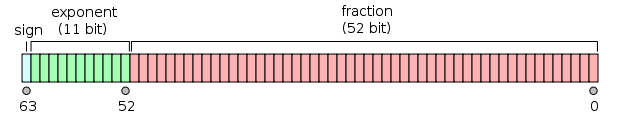@qidiandasheng 2022-08-09T14:46:22.000000Z 字数 4939 阅读 2185

# 浮点数精度问题（😁）

计算机系统

## 单精度浮点数和双精度浮点数

int type_size_float = sizeof(float) << 3;printf("float 字节数：%ld 位数%d",sizeof(float),type_size_float);printf("\n");int type_size_double = sizeof(double) << 3;printf("double 字节数：%ld 位数%d",sizeof(double),type_size_double);输出值：float 字节数：4 位数32double 字节数：8 位数64

（1）符号位（Sign）：0代表正数，1代表负数（2）指数位（Exponent）：用于存储科学计数法中的指数部分，并且采用移位存储(单精度：127+指数  双精度：1023+指数)的二进制方式。（3）尾数位（Mantissa）：用于存储尾数部分（单精度23(bit)，双精度52(bit)）

float的符号位，指数位，尾数分别为1, 8, 23。 如图：double的符号位，指数位，尾数分别为1, 11, 52。如图：IEEE754标准中，一个规格化浮点数x的真值表示为：

x=(−1)^s*(1.M)*2^e

### 例子解析

整数部分：除以2，商继续除以2，得到0为止，将余数逆序排列。22 / 2     11 余 011/2       5  余 15  /2      2  余 12  /2      1  余 01  /2      0  余 1得到22的二进制是10110小数部分：乘以2，取整，小数部分继续乘以2，取整，得到小数部分0为止，将整数顺序排列。0.8125x2=1.625 取整1,小数部分是0.6250.625x2=1.25   取整1,小数部分是0.250.25x2=0.5     取整0,小数部分是0.50.5x2=1.0      取整1,小数部分是0，得到0.8125的二进制是0.1101结果：十进制22.8125等于二进制10110.1101

S(符号位)：0
E(指数位)：11111010=125
M(尾数位): 00100000000000000000000

x=(-1)^0*(1.00100000000000000000000)*2^-2=0.28125

## 精度缺失问题

float a=0.1;printf("%.10f\n",a);float a2=0.2;printf("%.10f\n",a2);float a3=0.3;printf("%.10f\n",a3);float a4=0.4;printf("%.10f\n",a4);float a5=0.5;printf("%.10f\n",a5);float a6=0.6;printf("%.10f\n",a6);float a7=0.7;printf("%.10f\n",a7);float a8=0.8;printf("%.10f\n",a8);float a9=0.9;printf("%.10f\n",a9);输出：0.10000000150.20000000300.30000001190.40000000600.50000000000.60000002380.69999998810.80000001190.8999999762
float a=0.1;printf("%.7f\n",a);float a2=0.2;printf("%.7f\n",a2);float a3=0.3;printf("%.7f\n",a3);float a4=0.4;printf("%.7f\n",a4);float a5=0.5;printf("%.7f\n",a5);float a6=0.6;printf("%.7f\n",a6);float a7=0.7;printf("%.7f\n",a7);float a8=0.8;printf("%.7f\n",a8);float a9=0.9;printf("%.7f\n",a9);输出：0.10000000.20000000.30000000.40000000.50000000.60000000.70000000.80000000.9000000

## 问题

### double转NSNumber时精度缺失

for (double i = 0.01; i<100; i+=0.01) {        NSNumber *number = [NSNumber numberWithDouble:i];        if ([[number stringValue] length]>5) {            NSLog(@"NSNumber: %@",number);            NSLog(@"NSNumber string:%@",[number stringValue]);            //0.07  0.56  0.57            NSString *doubleString = [NSString stringWithFormat:@"%lf", i];            NSDecimalNumber *decNumber = [NSDecimalNumber decimalNumberWithString:doubleString];            NSLog(@"NSDecimalNumber: %@",decNumber);            NSLog(@"NSDecimalNumber string:%@",[decNumber stringValue]);            NSLog(@"\n");        }    }
2018-07-07 16:10:55.727827+0800 NumberTest[38798:4238675] NSNumber: 0.070000000000000012018-07-07 16:10:55.728016+0800 NumberTest[38798:4238675] NSNumber string:0.070000000000000012018-07-07 16:10:55.728268+0800 NumberTest[38798:4238675] NSDecimalNumber: 0.069999999999999992018-07-07 16:10:55.728425+0800 NumberTest[38798:4238675] NSDecimalNumber string:0.07

### 思考

double amount1 = 4551.44;double amount2 = 44551.44;printf("%.2f\n%.2f\n",amount1,amount2);printf("%.20f\n%.20f\n",amount1,amount2);NSLog(@"\n%@\n%@",@(amount1),@(amount2));NSLog(@"\n%@\n%@",@(amount1*100),@(amount2*100));NSString *string = [NSString stringWithFormat:@"%.lf",amount1*100];NSString *string2 = [NSString stringWithFormat:@"%.lf",amount2*100];NSLog(@"\n%@\n%@",string,string2);输出：#printf("%.2f\n%.2f\n",amount1,amount2);4551.4444551.44#printf("%.20f\n%.20f\n",amount1,amount2);4551.4399999999995998223344551.44000000000232830644#NSLog(@"\n%@\n%@",@(amount1),@(amount2));4551.4444551.44#NSLog(@"\n%@\n%@",@(amount1*100),@(amount2*100));455143.99999999994455144#NSLog(@"\n%@\n%@",string,string2);4551444455144

## 参考• 私有
• 公开
• 删除